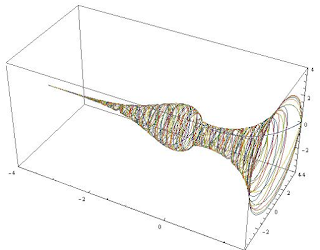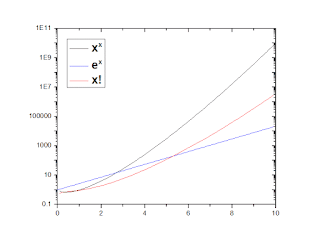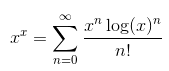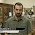Sunday, 22 November 2015

The Sophomore's Spindle: All about the function x^x

$x^x$. x-to-the-power-of-x. For natural numbers, it grows as 1, 4, 27, 256, 3125. It is not the most important or useful function, but it has a few cool properties, and I'll discuss them here. It is mostly known for growing really fast, but when plot as a complex function over negative numbers it has a cool shape. A special integral of this function is known as the "Sophomore's Dream," and in one of my ill-fated math attempts around 2010, I attempted to generalize that and find its anti-derivative. There isn't much centralized information about the $x^x$ function, so I hope to compile here in this blog post.The $x^x$ spindle, discussed below. Image source.

Other forms and names

It is generally hard to search for information about this function because the results include any time the letter x appears twice in succession. The name "self-exponential function" returns some results.

If repeated addition is multiplication, and repeated multiplication is exponentiation, repeated exponentiation is called tetration. The notation is a flipped version of exponentiation: $x^x$=$^{2}x$. So, our function here could be called second-order tetration. This also continues the property* of the number 2 that $^{2}2=2^{2}=2\times 2=2+2$.

The other common way to represent this function is as an exponential, rewriting it as $e^{\log x^x}=e^{x\log x}$. This makes it much easier to manipulate, because now only the exponent is variable rather than both the base and the exponent.

Growth

As can be seen in the numbers above, this function grows really fast, more than an order of magnitude per integer increase, which is just a way of saying it grows faster than the exponential function (because the base also increases), and is greater than the factorial of any natural number.This function grows really fast.

In the negative: the $x^x$ spindle.

The function can be calculated easily for positive integers, and also for negative integers, over which the function rapidly decays. However, for negative non-integers, the function's output is not always real (a simple case, $(-0.5)^{-0.5}$ is purely imaginary). In fact, it is only real for negative x if x is a rational number whose denominator is odd. To figure out how to calculate this function for negative numbers, we'll go hyperbolic and then try using logarithms. The function $e^x$ can be written as cosh(x)+sinh(x), the sum of hyperbolic cosines and sines. That means we can write our function as:

$x^{x}=\cosh(x\log(x))+\sinh(x\log(x))$

The logarithm is not unambiguously defined for negative numbers, but by exploiting Euler's identity and some logarithm rules, we can write $\log(-y)=\log(-1)+\log(y)$ and $log(-1)=log(e^{\pi i})=\pi i$. Therefore, log(-y)=log(y)+$\pi i$. This is cheating a bit, because you can multiply $\pi i$ in that exponential by any odd integer and still satisfy Euler. This is merely the first choice of infinite possibilities, which we'll stick with for now. Anyway, this means that if x is negative, then we can rewrite our function again:

$x^{x}=\cosh(x\log(-x)+\pi i x)+\sinh(x\log(-x)+\pi i x)$

Now, we use the sum formulae for sinh and cosh, which are cosh(a+b)=cosh(a)cosh(b)+sinh(a)sinh(b) and sinh(a+b)=sinh(a)cosh(b)+sinh(a)cosh(b). We also remember that cosh(ix)=cos(x) and sinh(ix)=i sin(x). If we do this expansion, simplify, and group by realness, we find:

$x^{x}=(-x)^{x}\left(\cos(\pi x)+i\sin(\pi x) \right)$

So, what happens when we plot this function in the negative domain? Its absolute value generally gets smaller, while its real and imaginary parts oscillate with a period of 2. It is purely real for integers, and purely imaginary for half-integers. Another way to plot this would be as a single curve with real and imaginary y-axes, in which case this function would trace out a spiral.$x^x$ over negative numbers.

However, this assumes our basic choice of the negative logarithm. We have a whole family of choices. Mark Meyerson realized something interesting, that the functions for various choices of logarithm follow the same envelope function with different frequency, such that all of them together trace out the shape a vase (which he calls the $x^x$ spindle). As more and more values of the logarithm are added, the spindle gets filled out (see the first picture).

Inverse

There is no simple function that is the inverse of $x^x$. However, there is a special function that was essentially almost designed to be the inverse of this function. The Lambert W Function is defined such that x=W(x)e$^{W(x)}$. The inverse of $x^x$ is:

There are two branches of the W function, and the inverse of $x^x$ swaps over to the other branch below x=1/e, such that its inverse passes the vertical line test for each branch.

This I don't think is very interesting, it's basically saying "the function that inverts the self-exponential function is defined as the function that inverts the self-exponential function." I guess you could call it the xth root of x, which is not the same as $x^{1/x}$ in this case.

Derivative

When students are first learning calculus, they learn that the derivative of a power function $x^n$ is simply $nx^{n-1}$. They also learn that the derivative of an exponential function $n^x$ is proportional to itself, with the constant of proportionality being the natural logarithm of the base: $n^{x}log(n)$, with n=e being a special case. It is not immediately obvious which rule to apply to $x^x$, although the second one is closer to being correct.

If we rewrite the function $x^{x}=e^{x\log{x}}$, its derivative can be found with the chain rule. The first step in the differentiation just gets us $e^{x\log{x}}=x^x$, and that gets multiplied the derivative of $x\log{x}$ which from the product rule is $(1)\log{x} + (x)\frac{1}{x}=1+\log{x}$. Multiplying the derivative of the innie by the derivative of the outie, we find:By finding when this equals zero, we can find the minimum and turning point of the function. This is simply just log(x)=-1, x=1/e=0.367..., and the minimum value is 0.692... One thing about this derivative is that it increases faster than function itself, contrary to the derivative of a power function. The rate of change of the function is even more divergent than the function itself.

Integrals: The Sophomore's Dream

One of the most interesting aspects of this function crops up when you try to integrate it. It actually comes from the reciprocal cousin of the function, $x^{-x}$, but the same phenomenon applies to the function itself. It is the identity:There is no immediately obvious reason why that should be true, but it is (it converges to roughly 1.29). The name Sophomore's Dream is an extension of the "freshman's dream," that (a+b)$^n$=$a^{n}+b^{n}$. It was first proven by Bernoulli in 1697. There is a similar identity for regular $x^x$, which is not as neat:This is proven by expanding the function as a series**:To find the integral, each term is integrated individually. Wikipedia, the free encyclopedia, gives a decent proof of how to integrate these terms, both in modern notation (that involves gamma functions) as well as with Bernoulli's original method. It's important that the limits of integration are zero and one, because the log(1) term kills some of the extraneous nasty terms in the antiderivative. The main step in the termwise integration involves a change of variable that turns it into the integrand that leads to the factorial function.Around 2009 or 2010, I thought I was clever because I found a way to express the indefinite integral of the $x^x$ function, that could be evaluated at values besides zero and one. Basically it involved using something called the incomplete gamma function, which is related to the factorial, to express the integral of each Taylor term in a general form. My solution was:
However, somewhat like the inverse, this is almost tautological and doesn't add much nuance. Still, I think I was the first person to figure this out. I tried writing a paper and submitting it to the American Mathematical Monthly (which is not at all the right journal for this) and got a rejection so harsh I still haven't read it almost six years later. However, in 2014 some Spanish researchers wrote a similar paper about the self-exponential function, and they came to the same conclusion as me regarding the incomplete gamma functions. So, I'm glad somebody got it out there.

Something else that's kind of interesting involving integrals and this function: the area under $x^{-x}$ over all positive numbers is like 1.99. I'm not sure if that's a coincidence or not.

Applications

Basically none.

The most common place the $x^x$ term pops up is in the Stirling approximation to the factorial, which is useful in statistical mechanics and combinatorics. It also gives a sense of the relative magnitude of self-exponentiation and factorials: one is literally exponentially smaller than the other.

In graduate statistical mechanics, there was a question about a square box, an n x n grid, with a fluid in it. At each point x along the box, the fluid could be at some height between 0 and n. So, the degeneracy of total states this fluid could have was $n^n$.If the fluid must be continuous and touch the bottom, it can take on $5^5$ different configurations.
If anyone knows any other applications of this function, let me know.

So just to summarize, the function $x^x$ grows really fast as has a few cool properties, but overall isn't the most useful of functions.

*The solution is 4.

**I had originally erroneously called this a Taylor series.

1.Good post, but I would avoid calling the expansion in powers of x and log Taylor series. Taylor series are always power series and only exist for functions that are analytic at the central point. This is merely a uniformly converging asymptotic series, which is enough to exchange the order of summation and integration.

1.Yes you're right, it's not a Taylor series. My mistake.

2.I think you've made an error in the sinh sum formula. Switch the arguments in one of the terms.

3.Possible references from OEIS: A000312
https://oeis.org/A000312
Number of labeled mappings from n points to themselves,
Number of labeled pointed rooted trees on n nodes,
...

1.Guess I should read up on graph theory.